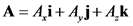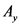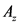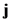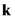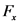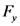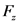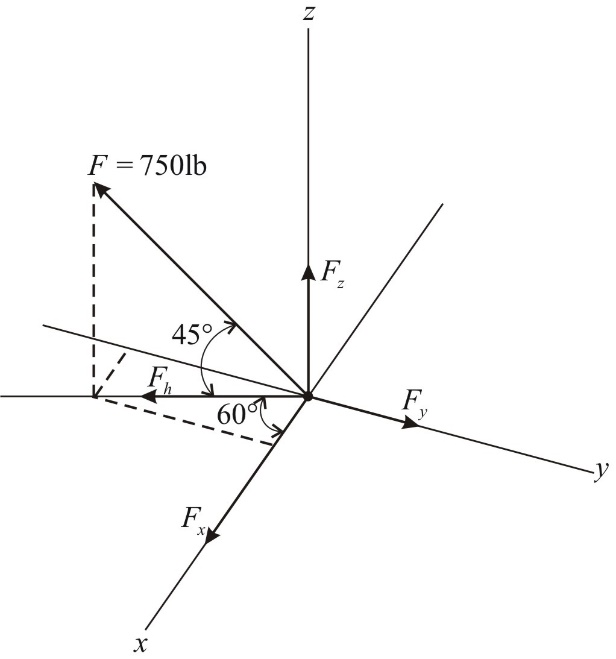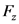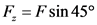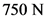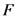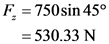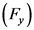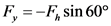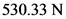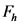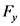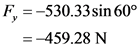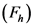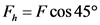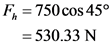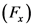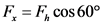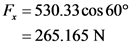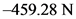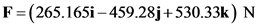# Express the force as a cartesian vector. (figure 1)Fundamental Problem 2.17 Part A Express the force as a Cartesian vector. (Figure 1) Express your answer in terms of the unit vectors i, j, k. To denote vectors in your answers, be sure to select the vec button.

General guidance

Concepts and reason
Cartesian vector representation:
Consider a vector A having components along x, y and z axes. Then the vector A can be written in Cartesian vector from as:

Here, , and are the components of vector A along x, y and z axes and , and are the unit vectors along x, y and z axes.
Cartesian vectors are used to simplify the vector algebra in three dimensions.

Fundamentals

The Cartesian form of the force F is,
……. (1)
Here, the components of the force vector along x, y and z Fare, and respectively.

Step-by-step

Step 1 of 3

Draw the free body diagram of the system as shown below.

After identifying the forces acting in the system, the free body diagram of the system is drawn as shown above.

Check for the directions of the force and the representation of forces along respective coordinate axis before drawing the free body diagram.

Calculate the components of force along each coordinate axis using the angles given in the free body diagram.

Step 2 of 3

Calculate the z component of the force using relation.

Substitute, for in relation above and calculate.

Calculate the y component of the force using relation.

Substitute, for in relation above and calculate.

Calculate horizontal component of the force on xy plane.

Substitute, for in relation above and calculate.

Calculate the x component of the force using relation.

Substitute, for in relation above and calculate.

The force along each coordinate axis are calculated as per the inclination made by the force F.

The inclination made by the force with the corresponding axis must be identified clearly. The common mistake that will happen is, considering only the inclination made by the force F neglecting the remaining angles.

Calculate the Cartesian vector form of force using the components of forces along each coordinate axis.

Step 3 of 3

Calculate the Cartesian vector form of force.
Using equation (1),

Substitute for, for, for.

The Cartesian vector form of force is.

The sum of the force components along each coordinate axis gives the Cartesian vector form of the given force.

The representation of the force must be done correctly. The positive and negative signs obtained for each force must be considered carefully.

The Cartesian vector form of force is.

The Cartesian vector form of force is.

A=&i+A;j+ĄK
F=F_i+F,j+F_K
N F = 750lb – – 607 –
F = Fsin 45°
750 N
F, = 750sin 45° = 530.33 N
F, =-F, sin 60°
530.33 N
F = -530.33 sin 60° = -459.28 N
F = F cos 45º
750 N
Fn = 750 cos 45° = 530.33 N
F = F, cos 60°
530.33 N
F = 530.33 cos 60° = 265.165 N
F=F_i+F,j+F_K
265.165 N
-459.28 N
530.33 N
F=(265.1651 – 459.28j+530.33k) N
(265.1651 – 459.28j+530.33k) N
(265.1651 – 459.28j+530.33k) N
We were unable to transcribe this image